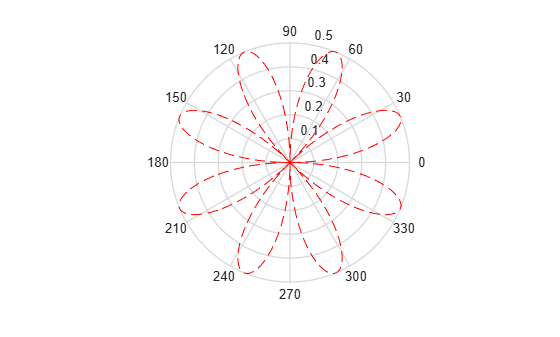# polar

(Not recommended) Polar coordinate plot

`polar` is not recommended. Use `polarplot` instead.

## Syntax

```polar(theta,rho) polar(theta,rho,LineSpec) polar(axes_handle,...) h = polar(...) ```

## Description

The `polar` function accepts polar coordinates, plots them in a Cartesian plane, and draws the polar grid on the plane.

`polar(theta,rho)` creates a polar coordinate plot of the angle `theta` versus the radius `rho`. `theta` is the angle from the x-axis to the radius vector specified in radians; `rho` is the length of the radius vector specified in dataspace units.

`polar(theta,rho,LineSpec)` `LineSpec` specifies the line type, plot symbol, and color for the lines drawn in the polar plot.

`polar(axes_handle,...)` plots into the axes with the handle `axes_handle` instead of into the current axes (`gca`).

`h = polar(...)` returns the line object in `h`.

## Examples

collapse all

Create a simple polar plot using a dashed red line.

```theta = 0:0.01:2*pi; rho = sin(2*theta).*cos(2*theta); figure polar(theta,rho,'--r')```## Tips

Negative `r` values reflect through the origin, rotating by `pi` (since `(theta,r)` transforms to `(r*cos(theta), r*sin(theta))`). If you want different behavior, you can manipulate `r` prior to plotting. For example, you can make `r` equal to `max(0,r)` or `abs(r)`.

## Version History

Introduced before R2006a